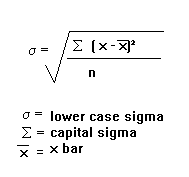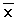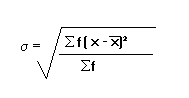## Define compound bar graph

A compound bar graph, also known as a stacked bar graph, is a type of graphical representation that displays multiple data sets using a series of horizontal or vertical bars. In a compound bar graph, each bar is divided into segments or sections that represent different categories or sub-groups within the data.

The main purpose of a compound bar graph is to compare the total values of different categories and observe the distribution or composition of each category within the whole. Each segment of the bar corresponds to a specific category, and the length or height of the segment represents the magnitude or proportion of that category within the total value.

Compound bar graphs are effective for illustrating both the individual values of different categories and the relationships between them. By stacking the segments on top of each other, the graph provides a visual representation of how the different categories contribute to the whole. This allows for easy comparisons and identification of patterns or trends within the data.

The compound bar graph is especially useful when comparing data sets that have a common measurement unit or when analyzing data over multiple time periods. It provides a clear visual representation of the relative sizes and proportions of each category and allows for easy interpretation of the data.

RELATED POSTS

## 5 ADVANTAGES OF A LINE GRAPH IN REPRESENTING STATISTICAL DATA

A line graph is a type of chart that uses lines to connect points to show how data changes over time. The points are usually plotted on a coordinate plane, with the x-axis representing the time or independent variable and the y-axis representing the data or dependent variable.

Line graphs are a versatile tool that can be used to visualize a wide variety of data. They are often used to track changes in population, economic indicators, weather patterns, and other phenomena.

## The following are advantages of line graph to represent statistical data

### Easy to draw

Line graphs are relatively easy to draw, even for people who are not familiar with graphing techniques. This makes them a good choice for people who want to create their own graphs to represent statistical data.

### Allow comparison

Line graphs can be used to compare two or more sets of data by plotting the data points for each set on the same graph. This makes it easy to see how the data sets are related to each other and to identify any trends or patterns.

### Easy to read and interpret

Line graphs are also easy to read and interpret. The data points are plotted on a grid, which makes it easy to see the values of the data and to identify any changes in the data over time. The lines connecting the data points can also be used to visualize trends or patterns in the data.

### Clear visual impression

Line graphs can create a clear visual impression of the data. This makes them a good choice for presentations or reports where you want to communicate the data in a way that is easy to understand.

### Can be used to show wide range of data

Line graphs can be used to show a wide range of data, from small changes to large changes. This makes them a versatile tool that can be used to represent a variety of statistical data.

### To show trends over time

Line graphs are a great way to show how data changes over time. This can be useful for tracking things like population growth, economic growth, or the spread of a disease.

For example, a line graph could be used to show the number of people living in Tanzania over time. The graph would start with the number of people in 1961 and then show how the number has changed each year since then. This would allow you to see the overall trend in population growth, as well as any spikes or dips.

### To show the relationship between two or more variables

Line graphs can also be used to show the relationship between two or more variables. This can be useful for understanding how different factors affect each other.

For example, a line graph could be used to show the relationship between the price of gasoline and the number of miles driven. The graph would show how the price of gasoline has changed over time, and how the number of miles driven has changed over time. This would allow you to see how the two variables are related to each other.

### To show comparisons between different groups of data

Line graphs can also be used to show comparisons between different groups of data. This can be useful for understanding how different groups are performing or how they are different from each other.

For example, a line graph could be used to show the average income of different countries over time. The graph would show how the average income has changed in each country, and how the countries compare to each other. This would allow you to see which countries are doing well economically and which countries are struggling.

### To show the distribution of data

Line graphs can also be used to show the distribution of data. This can be useful for understanding how data is spread out and where the most common values are.

For example, a line graph could be used to show the distribution of heights in a population. The graph would show how many people are of each height, and how the heights are distributed. This would allow you to see what the average height is and how many people are taller or shorter than average.

### To show the impact of changes in one variable on another variable

Line graphs can also be used to show the impact of changes in one variable on another variable. This can be useful for understanding how one factor affects another.

For example, a line graph could be used to show the impact of a new policy on the number of people who are unemployed. The graph would show how the number of unemployed people has changed since the policy was implemented. This would allow you to see whether the policy has had the desired effect.

These are just a few of the advantages of using a line graph in representing statistical data. Line graphs are a versatile tool that can be used to visualize data in a variety of ways. When used correctly, they can be a powerful tool for understanding and communicating data.

## Here are some additional things to keep in mind when using line graphs:

• Choose the right scale: The scale of the line graph should be appropriate for the data that you are plotting. If the scale is too small, the changes in the data will be difficult to see. If the scale is too large, the graph will be cluttered and difficult to read.
• Use clear labels: The labels on the axes of the line graph should be clear and concise. They should also be consistent with the units of measurement that are being used.
• Use a legend: If you are plotting multiple lines on the same graph, you should use a legend to identify each line. This will help the reader to understand what each line represents.
• Use a title: The title of the line graph should be clear and concise. It should also accurately describe the data that is being plotted.

RELATED POSTS

## QUESTION ONE

Data in the following table show the enrolment of form one student at mji mpya secondary school from 2006 to 2010. Carefully study  them and answer the questions that follow

(a)    Present the data by using a simple bar graph

(b)   Comment on the trend of enrolment

(c)  Explain the advantages of the methods used in (a) above

## QUESTION TWO

State the difference between the following types of data

(a) Discrete and continuous

(b)   Single and grouped

## QUESTION THREE

Read carefully the hypothetical data presented below and then answer the questions that follow. Cash crops production in Tanzania in ‘000’ tons

(a)    Represent data above using a compound bar graph

(b) How the compound bar graph is of value to you? Give three points

(c)  Comment on the trend of production as observed from the graph

## QUESTION FOUR

Read carefully the climatic data for station A presented in the table and answer the question that follow

I. Describe the climatic condition of station A

II.      Name any two countries in the great lakes of East Africa that experience this type of climatic condition

III.  Present the data graphically

## QUESTION FIVE

Study the following data showing the production of Irish potatoes  in thousands (‘000’) tonnes in three villages in Tanzania from 2000 to 2002 then answer the question that follows

(a)  Construct a compound bar graph to show the production of Irish potatoes in the three villages

(b)   Give three advantages and two disadvantages of the compound bar graph

(c) Suggest any other three methods which could be used to present the data provided in the table

## QUESTION SIX

Classify the nature of geographical data

II.   The geography teacher of mtakuja secondary school obtained the following scores after marking the annual examinations of his students; 36,43,48,68,70,19,49,30,51,18,51,58,72,51,61,41,51,26

a)  Find the median of the score

b)  Calculate the arithmetic mean

c)  Determine the mode of the score

III.   Show the significance of the arithmetic mean in geographic data

## QUESTION SEVEN

Carefully study the hypothetical data provided below showing the exports of crops from Tanzania. The exports of crops in tonnes

(a)    Present the data using compound bar graph (b)   Explain two advantages and disadvantages of compound bar graph

## QUESTION EIGHT

(a)    Explain the meaning of standard deviation

(b)   Study the following data showing the age of primary school pupils at tumaini primary school and answer the question that follow; 15,8,7,6,12,5,14 and 13

I.     Determine the range and median of the age of students

II.  Calculate the standard deviation

## QUESTION NINE

The table below shows agricultural production in Tanzania from 2000 to 2003 in thousands (‘000’) tons

(a)    Present the data by using grouped bar graph (b)   Comment on the nature of production

## HOW TO CALCULATE STANDARD DEVIATION FOR GROUPED AND UNGROUPED DATA

Non-Grouped Data
Non-grouped data is just a list of values. The standard deviation is given by the formula:s means ‘standard deviation’.
S means ‘the sum of’.means ‘the mean’

Example
Find the standard deviation of 4, 9, 11, 12, 17, 5, 8, 12, 14
First work out the mean: 10.222

Now, subtract the mean individually from each of the numbers given and square the result.

This is equivalent to the (x –)² step. x refers to the values given in the question.

Now add up these results (this is the ‘sigma’ in the formula): 139.55
Divide by n. n is the number of values, so in this case, is 9. This gives us: 15.51
And finally, square root this: 3.94

The standard deviation can usually be calculated much more easily with a calculator and this may be acceptable in some exams. On my calculator, you go into the standard deviation mode (mode ‘.’).

Then type in the first value, press ‘data’, type in the second value, press ‘data’.

Do this until you have typed in all the values, then press the standard deviation button (it will probably have a lower case sigma on it). Check your calculator’s manual to see how to calculate it on yours.

NB: If you have a set of numbers (e.g. 1, 5, 2, 7, 3, 5 and 3), if each number is increased by the same amount (e.g. to 3, 7, 4, 9, 5, 7 and 5), the standard deviation will be the same and the mean will have increased by the amount each of the numbers was increased by (2 in this case).

This is because the standard deviation measures the spread of the data. Increasing each of the numbers by 2 does not make the numbers any more spread out, it just shifts them all along.

Grouped Data
When dealing with grouped data, such as the following:

the formula for standard deviation becomes:Try working out the standard deviation of the above data. You should get an answer of 1.32 .
You may be given the data in the form of groups, such as:

In such a circumstance, x is the midpoint of groups.

## arithmetic mean

arithmetic mean is the average of all values in a set of distribution.

it is determined by adding up all values and dividing by the sum of observations added.

arithmetic mean is used to assess the distribution value whether was high or low

### advantages of the arithmetic mean

• it is easy to calculate and the majority of people understand it
• it is used to check the values if high or low
• it can be used for further calculation; for example arithmetic mean is used to calculate standard deviation

• arithemetic mean has the big weakness of being pulled toward an outlier (extreme score)
• it need high mathematical knowledge to calculate arithematic mean for grouped data

## 2 CLASSIFICATION OF STATISTICS

Statistics being the scientific and systematic methods dealing with numerical facts is broadly categorized into two types depending on how data is handled.

The two main categories of statistics are descriptive and inferential statistics.

## Descriptive statistics

this deals with recording, summarizing, analyzing and presentation of numerical facts that have been actually collected.

Descriptive statistics is the term given to the analysis of data that helps describe, show or summarize data in a meaningful way such that, for example, patterns might emerge from the data.

Descriptive statistics do not, however, allow us to make conclusions beyond the data we have analyzed or reach conclusions regarding any hypotheses we might have made.

They are simply a way to describe our data. Descriptive statistics are very important because if we simply presented our raw data it would be hard to visualize what the data was showing, especially if there was a lot of it.

Descriptive statistics, therefore, enables us to present the data in a more meaningful way, which allows a simpler interpretation of the data.

For example, if we had the results of 100 pieces of students’ coursework, we may be interested in the overall performance of those students.

We would also be interested in the distribution or spread of the marks. Descriptive statistics allow us to do this.

Typically, there are two general types of statistics that are used to describe data they are a measure of central tendency and measure of spread

Inferential statistics

this makes inferences about populations using data drawn from the population.

Instead of using the entire population to gather the data, the statistician will collect a sample or samples from the millions of residents and make inferences about the entire population using the sample.

With inferential statistics, you are trying to reach conclusions that extend beyond the immediate data alone.

For instance, we use inferential statistics to try to infer from the sample data what the population might think.

Or, we use inferential statistics to make judgments of the probability that an observed difference between groups is a dependable one or one that might have happened by chance in this study.

Thus, we use inferential statistics to make inferences from our data to more general conditions; we use descriptive statistics simply to describe what’s going on in our data.

## ACSEE NECTA 2014 GEOGRAPHY PAPER ONE PAST PAPER

1. a)   differentiate the following statistical concept;
•        Inferential statistics and descriptive statistics

b) the form five students’ scores in geography subject at Nganza s.s were as follows

•  Calculate standard deviation
•   What are disadvantages of grouped data?

2. a) Explain the types of vertical aerial photographs

b) Describe why aerial photographs appear difficult in interpretation.

a)      distinguish the following research concepts ·

• research proposal and research report. ·
• Qualitative research and quantitative research ·
• Data collection and data analysis ·
• Population and sample

3. Describe five causes of plate tectonics movement and prove its existence by providing four pieces of evidence.

4. Explain the values of volcanism for the development of the society. Give eight points.

5. To what extent is river basin development both advantageous and disastrous?

6. Describe the factors that control the global distribution of plant communities.

7. Analyses six properties to be considered when studying soil profile at the field